# Copy Propagation and Common Subexpression Elimination in Titanium

• Slides: 22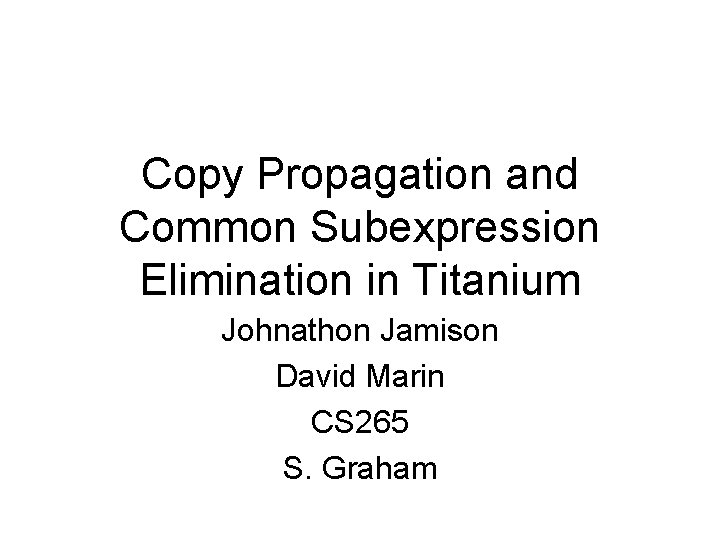Copy Propagation and Common Subexpression Elimination in Titanium Johnathon Jamison David Marin CS 265 S. Graham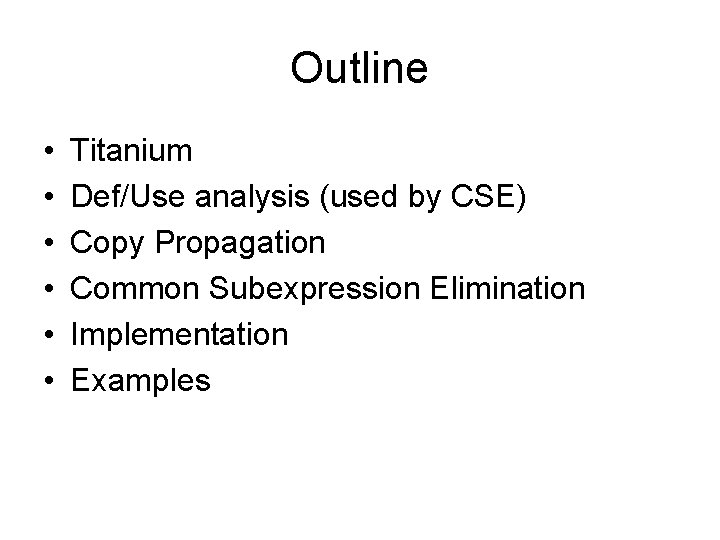Outline • • • Titanium Def/Use analysis (used by CSE) Copy Propagation Common Subexpression Elimination Implementation ExamplesTitanium • Titanium is an extension of Java • The Titanium compiler compiles Titanium code to C • The C code is then compiled by the system compiler, e. g. gcc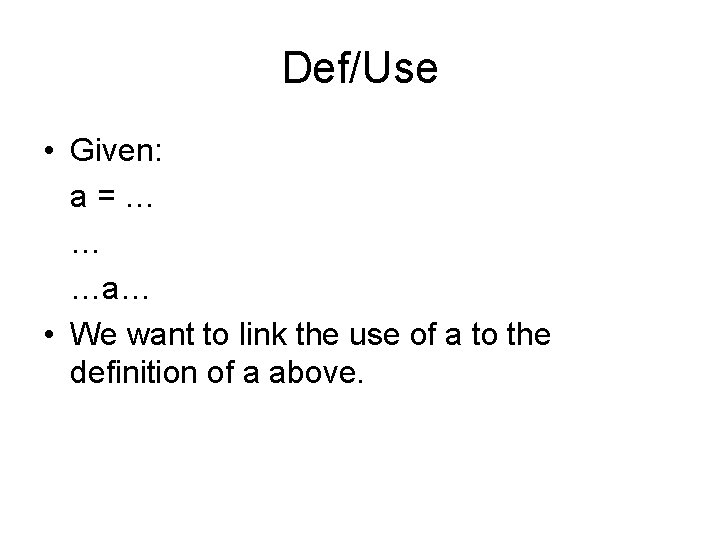Def/Use • Given: a=… … …a… • We want to link the use of a to the definition of a above.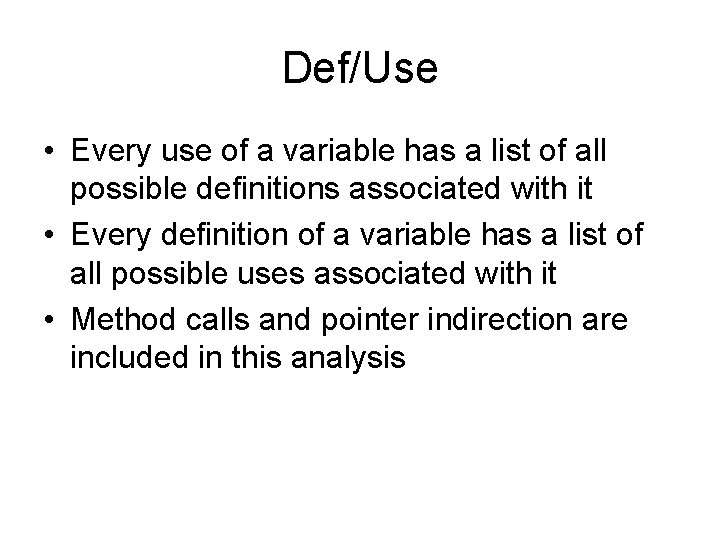Def/Use • Every use of a variable has a list of all possible definitions associated with it • Every definition of a variable has a list of all possible uses associated with it • Method calls and pointer indirection are included in this analysis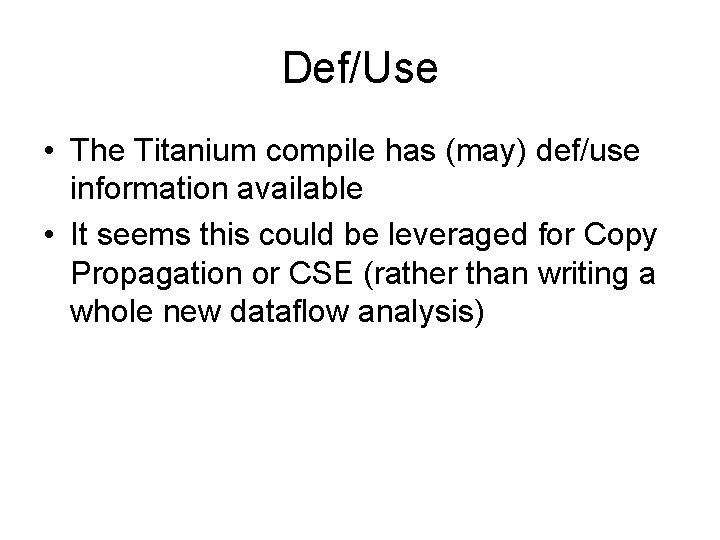Def/Use • The Titanium compile has (may) def/use information available • It seems this could be leveraged for Copy Propagation or CSE (rather than writing a whole new dataflow analysis)Global Copy Propagation • Given a = b; … x = a + 1; • We want to replace a with b on the last line, but we need to know that a and b are unchanged • Def/use analysis isn’t quite enough (why? )Inserting Fake Defs and Uses • Add fake defs and uses so that def/use analysis gives us the info we need b = b; a = b; … newfaketemp = b; x = a + 1; • We can use a similar technique to enable CSE.CSE • Given: a=f*i … b=f*i • We want to compute f * i only once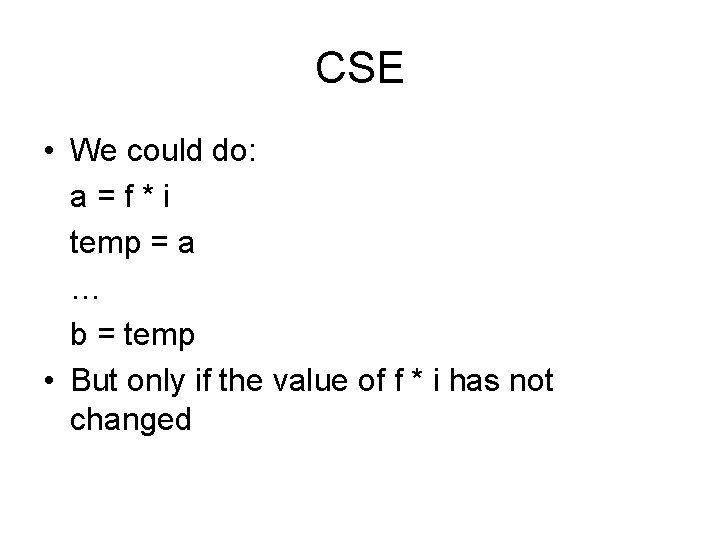CSE • We could do: a=f*i temp = a … b = temp • But only if the value of f * i has not changed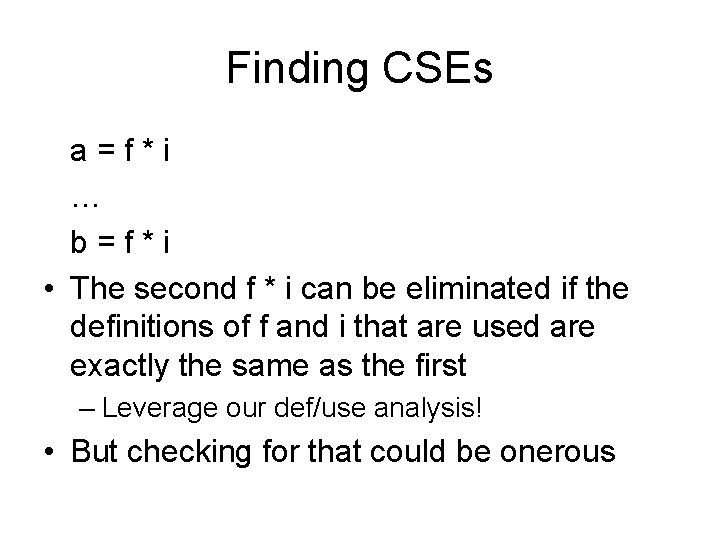Finding CSEs a=f*i … b=f*i • The second f * i can be eliminated if the definitions of f and i that are used are exactly the same as the first – Leverage our def/use analysis! • But checking for that could be onerous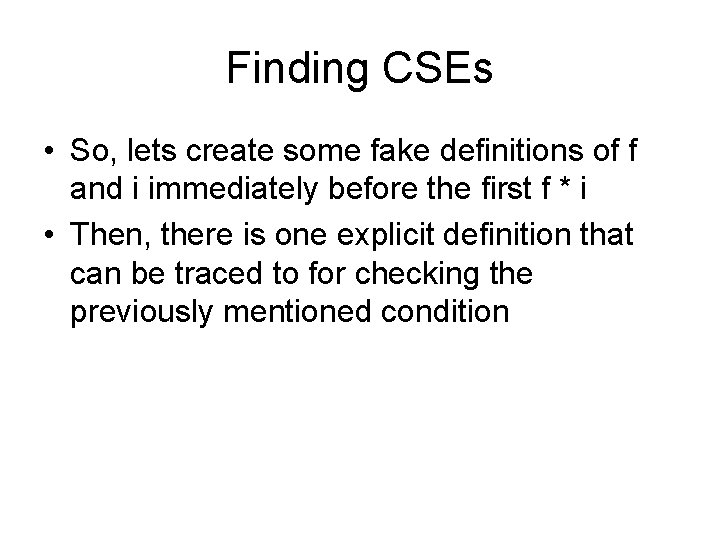Finding CSEs • So, lets create some fake definitions of f and i immediately before the first f * i • Then, there is one explicit definition that can be traced to for checking the previously mentioned conditionFinding CSEs f=f i=i a=f*i … b=f*i • Thus, if f and i have the same definitions in both places, then the second f * i can be eliminatedHanding Global CSEs • This is fine and dandy for straight line code, but what if you have: a=f*i b=f*i … … c=f*i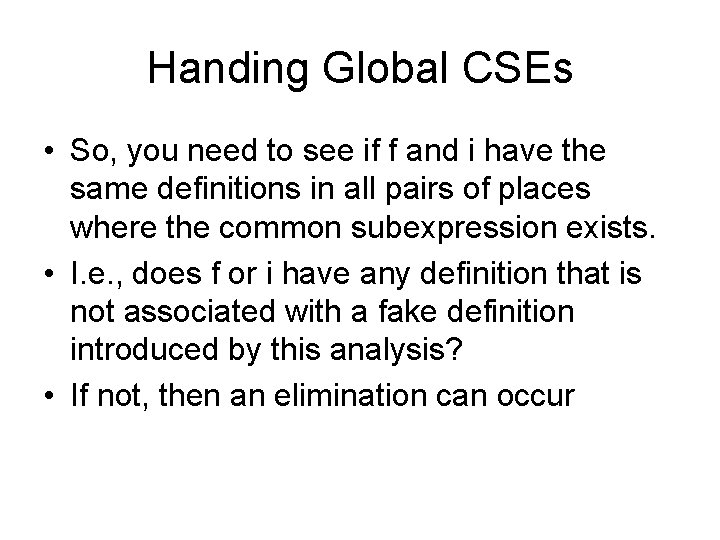Handing Global CSEs • So, you need to see if f and i have the same definitions in all pairs of places where the common subexpression exists. • I. e. , does f or i have any definition that is not associated with a fake definition introduced by this analysis? • If not, then an elimination can occur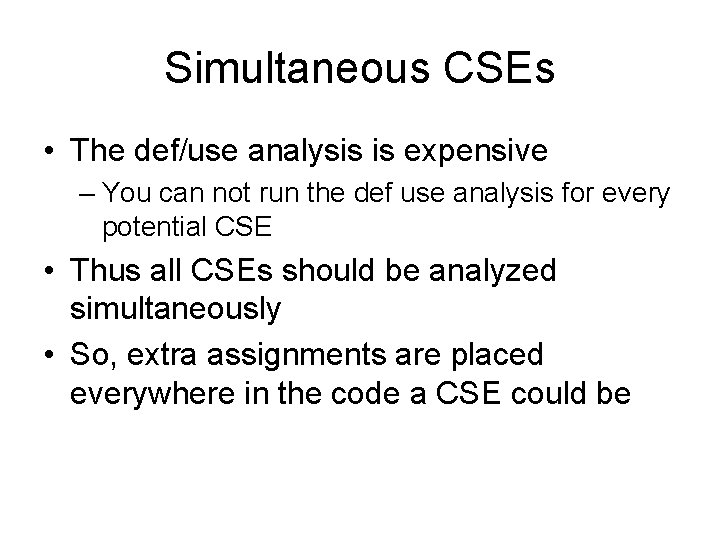Simultaneous CSEs • The def/use analysis is expensive – You can not run the def use analysis for every potential CSE • Thus all CSEs should be analyzed simultaneously • So, extra assignments are placed everywhere in the code a CSE could be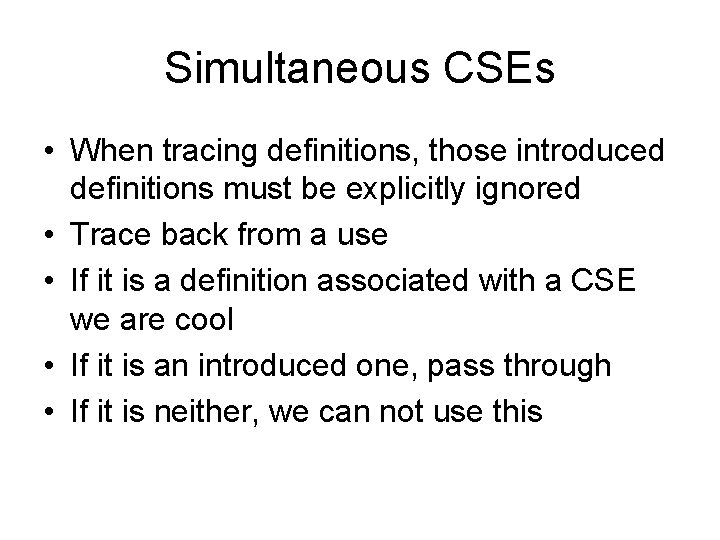Simultaneous CSEs • When tracing definitions, those introduced definitions must be explicitly ignored • Trace back from a use • If it is a definition associated with a CSE we are cool • If it is an introduced one, pass through • If it is neither, we can not use thisAltogether Now… • Insert the extra assignments • For every similar expression – At every site, try to eliminate this expression • Delete the assignments, so as not to interfere with anything elseInteraction with Copy Propagation • Any temps introduced are placed after the calculation, so that copy propagation can remove them a=f*i temp_1 = a … … b=f*i b = temp_1 temp_2 = b … … c=f*i c = temp_2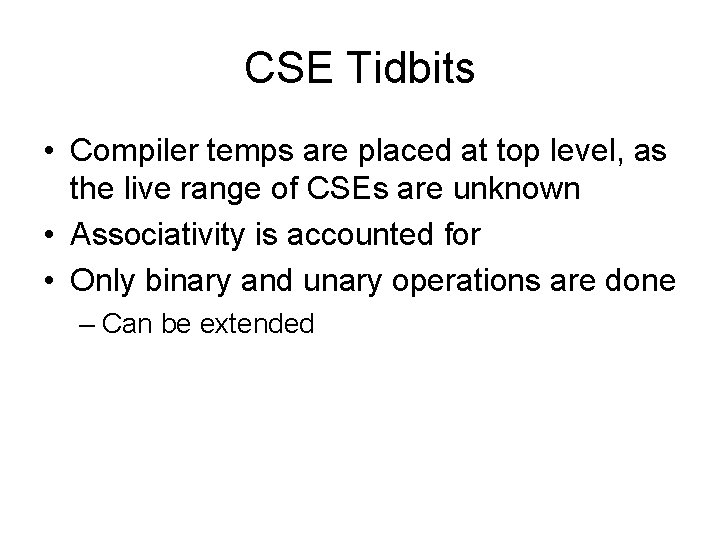CSE Tidbits • Compiler temps are placed at top level, as the live range of CSEs are unknown • Associativity is accounted for • Only binary and unary operations are done – Can be extendedExamples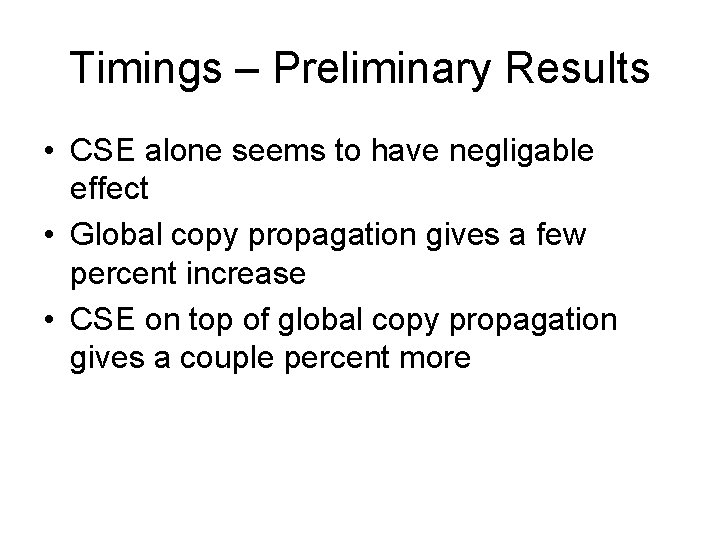Timings – Preliminary Results • CSE alone seems to have negligable effect • Global copy propagation gives a few percent increase • CSE on top of global copy propagation gives a couple percent more